O 1 O O 1 O O 1 O 1 1 O 1 1 O 1 O 1 1 O O O O 1 O 1 1 O O 1 1 1 O 1 1 O 1 O O 1 O 1 1 O 1 1 1 O O 1 1 O O O O 1 O 1 1 1 O 1 O O O 1 1 O 1 O O 1 O 1 1 O 1 1 1 1 O 1 1 O 1 1 1 O O O 1 O O O O O O 1 1 O 1 O O 1 O 1 1 1 O O 1 1 O O 1 O O O O O O 1 1 O 1 1 O 1 O 1 1 O 1 1 1 1 O 1 1 1 O O 1 O O 1 1 O O 1 O 1 O O 1 O O O O O O 1 1 O 1 O O 1 O 1 1 O 1 1 O 1 O 1 1 1 O O O O O 1 1 O 1 1 1 1 O 1 1 1 O O 1 O O 1 1 1 O 1 O O O 1 1 O O O O 1 O 1 1 O 1 1 1 O O 1 1 1 O 1 O O O O 1 O O O O O O 1 1 1 O 1 O O O 1 1 O 1 O O O O 1 1 O O O O 1 O 1 1 O 1 1 1 O O O 1 O O O O O O 1 1 O 1 O 1 1 O 1 1 O 1 1 1 O O 1 1 O 1 1 1 1 O 1 1 1 O 1 1 1 O 1 1 O 1 1 O O O 1 1 O O 1 O 1 O 1 1 O O 1 O O O 1 1 O O 1 1 1 O 1 1 O O 1 O 1 O O 1 O 1 1 1 O O O 1 O O O O O O 1 O O O 1 1 O O 1 1 O 1 1 1 1 O 1 1 1 O O 1 O O O 1 O O O O O O 1 1 O 1 O 1 1 O 1 1 O 1 1 1 O O 1 1 O 1 1 1 1 O 1 1 1 O 1 1 1 O 1 1 O 1 1 O O O 1 1 O O 1 O 1 O 1 1 O O 1 O O O 1 1 O O 1 1 1 O 1 1 O O 1 O 1 O O 1 O O O O O O 1 1 O 1 O O 1 O 1 1 1 O O 1 1 O O 1 O O O O O O 1 1 O 1 1 O O O 1 1 O 1 O O 1 O 1 1 O 1 1 O 1 O 1 1 O 1 O O 1 O 1 1 1 O 1 O O O 1 1 O O 1 O 1 O 1 1 O O 1 O O O O 1 O O O O O O 1 1 1 O 1 O O O 1 1 O 1 1 1 1 O O 1 O O O O O O 1 1 O O O O 1 O 1 1 O 1 1 O O O 1 1 O 1 1 O O O O 1 O O O O O O 1 1 1 O 1 1 1 O 1 1 O O 1 O 1 O O 1 O O O O O O 1 1 O 1 1 1 O O 1 1 O 1 1 1 1 O 1 1 1 O 1 1 1 O O 1 O O O O O O 1 1 O 1 O 1 1 O 1 1 O 1 1 1 O O 1 1 O 1 1 1 1 O 1 1 1 O 1 1 1 O O 1 O O O O O O 1 1 O O O O 1 O 1 1 O 1 1 1 O O 1 1 O O 1 O O O O 1 O O O O O O 1 1 1 O 1 O 1 O 1 1 O 1 1 1 O O 1 1 O O 1 O O O 1 1 O O 1 O 1 O 1 1 1 O O 1 O O 1 1 1 O O 1 1 O 1 1 1 O 1 O O O 1 1 O O O O 1 O 1 1 O 1 1 1 O O 1 1 O O 1 O O O O 1 O 1 1 O O O O 1 O O O O O O 1 1 1 O 1 1 1 O 1 1 O 1 O O O O 1 1 O 1 O O 1 O 1 1 O 1 1 O O O 1 1 O O 1 O 1 O O 1 O O O O O O 1 1 O 1 O O 1 O 1 1 O 1 1 O 1 O 1 1 O O O O 1 O 1 1 O O 1 1 1 O 1 1 O 1 O O 1 O 1 1 O 1 1 1 O O 1 1 O O O O 1 O 1 1 1 O 1 O O O 1 1 O 1 O O 1 O 1 1 O 1 1 1 1 O 1 1 O 1 1 1 O O O 1 O O O O O O 1 1 O O 1 O 1 O 1 1 O 1 1 O 1 O 1 1 O O O 1 O O 1 1 1 O O 1 O O 1 1 O O O O 1 O 1 1 O O O 1 1 O 1 1 O O 1 O 1 O 1 1 1 O O 1 1 O O 1 O O O O O O 1 1 1 O 1 O O O 1 1 O 1 O O O O 1 1 O O 1 O 1 O O 1 O O O O O O 1 1 O O 1 O 1 O 1 1 O 1 1 1 O O 1 1 1 O 1 O O O 1 1 O 1 O O 1 O 1 1 1 O O 1 O O 1 1 O O 1 O 1 O O 1 O O O O O O 1 1 1 O 1 1 1 O 1 1 O 1 1 1 1 O 1 1 1 O O 1 O O 1 1 O 1 1 O O O 1 1 O O 1 O O O O 1 O 1 1 O O O O 1 O O O O O O 1 1 O O O O 1 O 1 1 O 1 1 1 O O 1 1 O O 1 O O O O 1 O O O O O O 1 1 O O O O 1 O 1 1 O 1 1 O O O 1 1 O 1 1 O O O O 1 O O O O O O 1 1 1 O 1 O O O 1 1 O 1 O O O O 1 1 O O 1 O 1 O 1 1 1 O O 1 O O 1 1 O O 1 O 1 O O 1 O O O O O O 1 1 O O 1 O 1 O 1 1 1 O 1 1 O O 1 1 O O 1 O 1 O 1 1 1 O O 1 O O O 1 O O O O O O 1 1 1 O 1 1 1 O 1 1 O 1 O O 1 O 1 1 O 1 1 O O O 1 1 O 1 1 O O O O 1 O O O O O O 1 1 O O O 1 O O 1 1 O O 1 O 1 O O 1 O O O O O O 1 1 1 O 1 O O O 1 1 O 1 1 1 1 O O 1 O O O O O O 1 1 O 1 O 1 1 O 1 1 O 1 1 1 O O 1 1 O 1 1 1 1 O 1 1 1 O 1 1 1 O O 1 O O O O O O 1 1 O O O O 1 O 1 1 O 1 1 1 O O 1 1 O O 1 O O O O 1 O O O O O O 1 1 1 O 1 O 1 O 1 1 O 1 1 1 O O 1 1 O O 1 O O O 1 1 O O 1 O 1 O 1 1 1 O O 1 O O 1 1 1 O O 1 1 O 1 1 1 O 1 O O O 1 1 O O O O 1 O 1 1 O 1 1 1 O O 1 1 O O 1 O O
Video 2 Photo - HD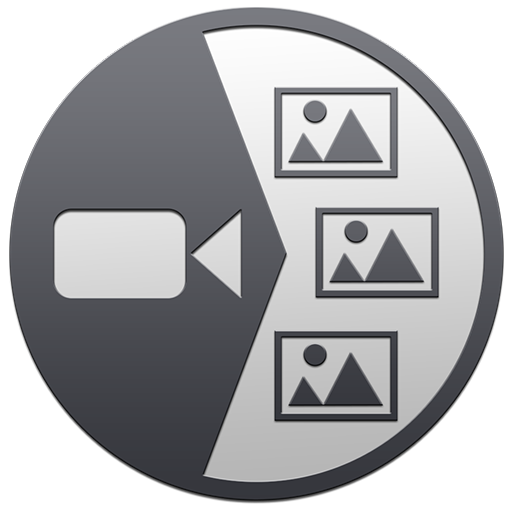# 介绍

Video 2 Photo - HD提供了一种快速、有趣和原始的方式从视频（和Live Photos）导出一张或多张照片。

• 有时你可能犹豫是拍照还是录像。最后决定录像!幸亏有了了'Video 2 Photo',现在可从视频抽取出许多图片来!
• 在想拍照时无意中录了像。使用'Video 2 Photo',可最终从此视频恢复照片。
• 拍摄好的移动物体、动物或人的照片几乎不可能。使用'Video 2 Photo'可实现。
• 拍摄一张自己的照片总是很困难。使用'Video 2 Photo',你可选择一张最适合你的好图片。
• 您需要一个精确的工具来导出多个连续照片并将其与其他应用程序一起使用。
• 从你最喜欢的视频中选择一张代表性图片作为墙纸或打印出来随身收藏。
• 一帧一帧地观看你的收藏影片有趣极了,可发现从未见到的小细节。

# 视频

Drag & Drop
 Mainly Landscape Batch Export

# Mac截图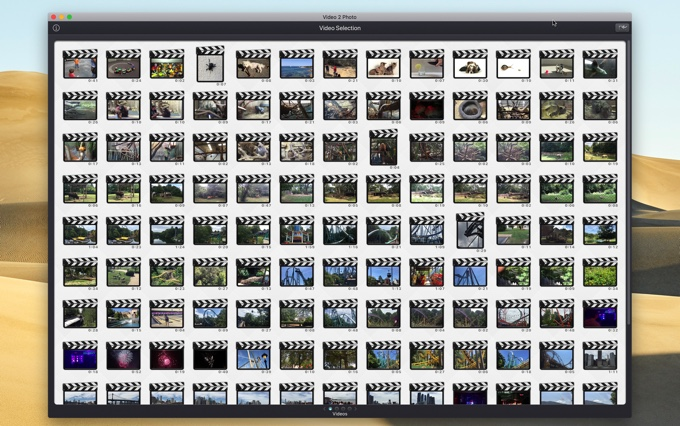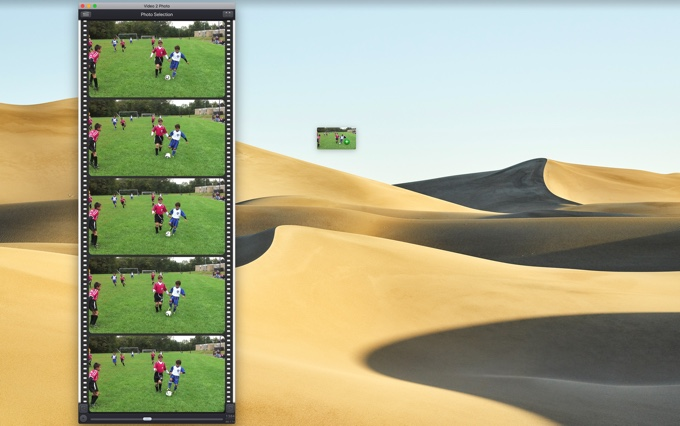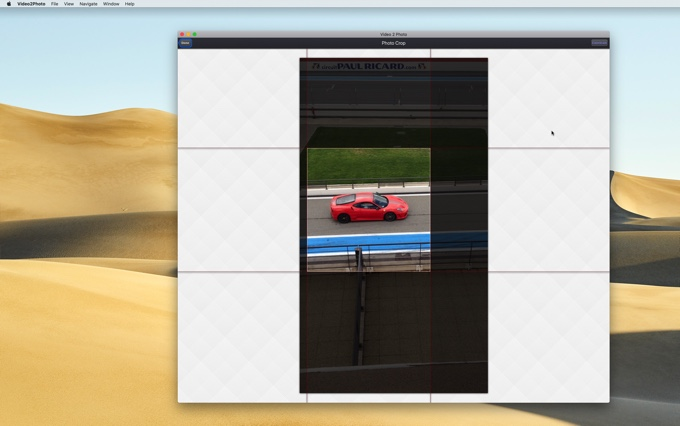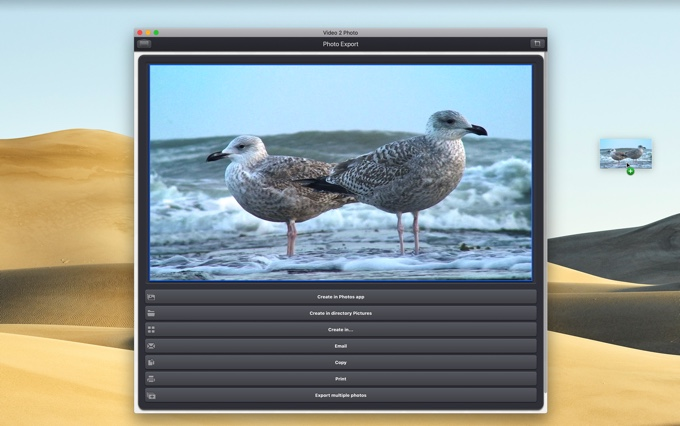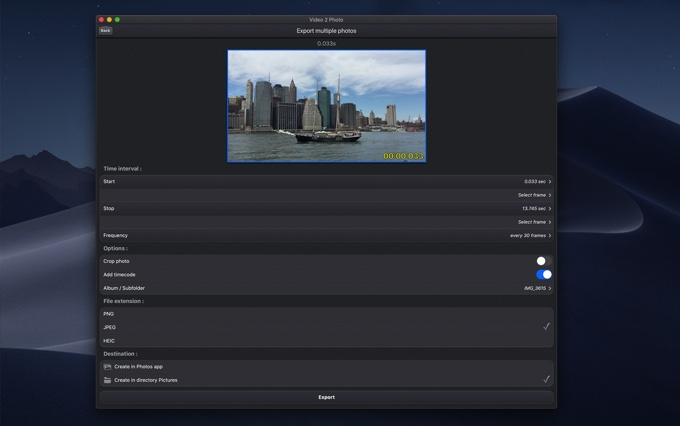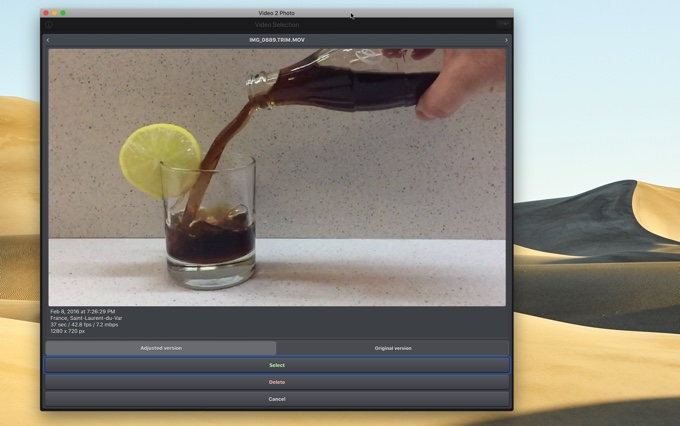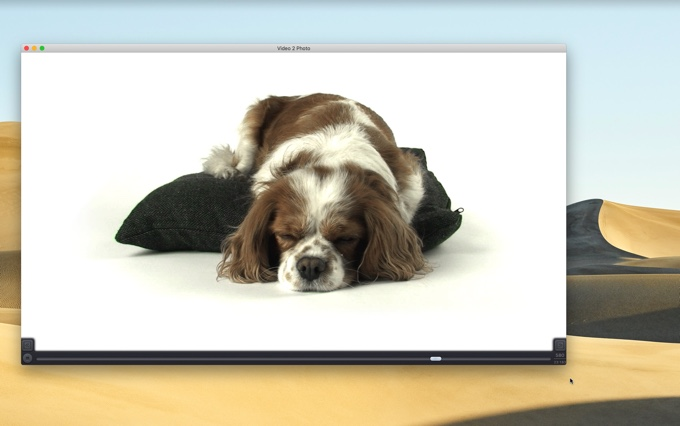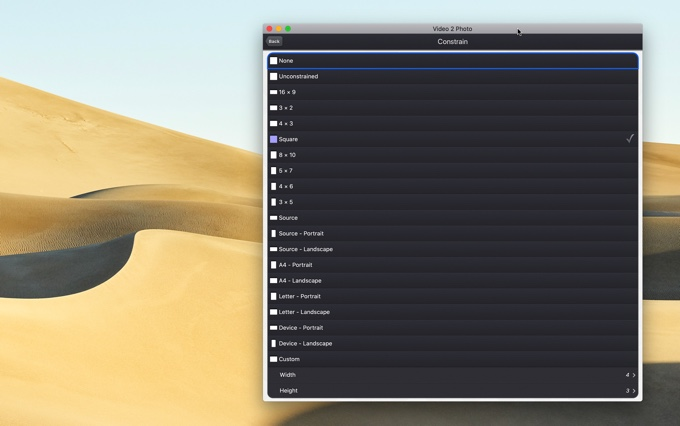• 小修复和改进。

# 可用性